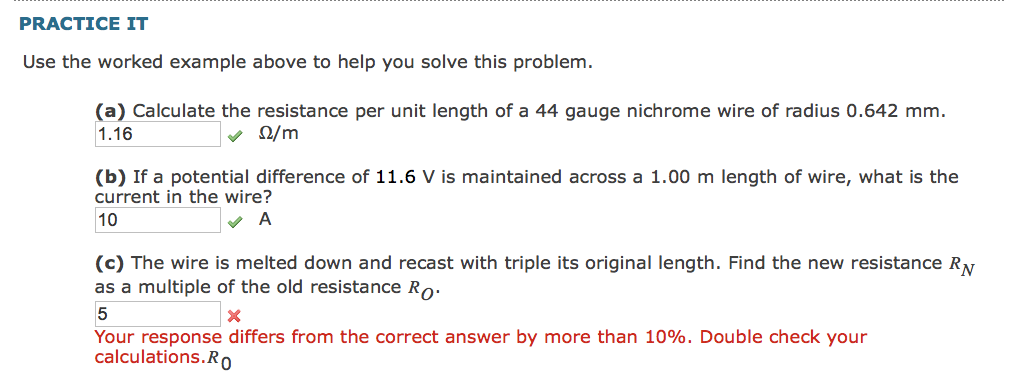# Question Just need the correct answer and explanation for c. Thanks PRACTICE IT Use the worked example above to help you solve this problem. (a) Calculate the resistance per unit length of a 44 gauge nichrome wire of radius 0.642 mm. 1.16 2/m (b) If a potential difference of 11.6 V is maintained across a 1.00 m length of wire, what is the current in the wire? 10 A (c) The wire is melted down and recast with triple its original length. Find the new resistance RN as a multiple of the old resistance Ro 5 x Your response differs from the correct answer by more than 10%. Double check your calculations.RO